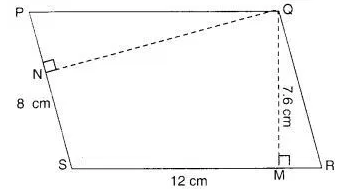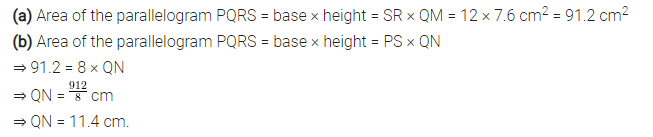# PQRS is a parallelogram (in Figure). QM is the height from Q to SR and

PQRS is a parallelogram (in Figure). QM is the height from Q to SR and QN is the height from Q to PS. If SR = 12 cm and QM =7.6 cm. Find :
(a) the area of the parallelogram PQRS
(b) QN, if PS = 8 cm.